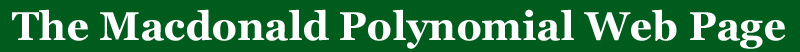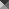BACK TO:Maple functions for computing
Macdonald polynomialsSF
Main functions:This is an integrated package for studying symmetric functionsIncludes limited functions for handling partitions

SF updated and should work on all versions of Maple.
John StembridgeACE
Main functions:This is a complete sytem for studying algebraic combinatoricsSymmetric Functions on different Alphabets, Free Module over symmetric polynomials, NonCommutative Symmetric  FunctionsCharacters of Linear Groups, Schubert Polynomials, SYMmetric Functions, TABleaux, FREE algebra, hyperoctahedral AlgebrasSymmetric Group Algebra, NilCoxeter Algebra, IDCoxeter Algebra, HEcKe Algebra, hyperoctahedral groups, Symmetric GroupsClassical Groups, PARTitions, COMPositionsMacdonald.html
Main functions:defintions of Macdonald polynomials P_\mu[X;q,t], J_\mu[X;q,t], H_\mu[X;q,t]Vertex operators for J & H basis to add row and column of size 2Vertex operators for J & H that add a row of size 3 to a polynomial indexed by a two column shapeFunctions to compute J & H basis that are FAST when the case is covered by the vertex operator formulasHall-Littlewood SFs and vertex operator

Vertex operator formulas are from:

M. A. Zabrocki, A Macdonald Vertex Operator and
Standard Tableaux Statistics for the Two-Column $(q,t)$-Kostka Coefficients,
Electron. J. Combinat. 5, R45 (1998), 46pp.JackoMaco.html
Main functions:Functions for creating symmetric and non-symmetric Jack and Macdonald symmetric functionsCherednick's double affine Hecke algebra functions

Provided by A. LascouxDeterm.html
Main functions:Determinental formulas for the Macdonald polynomials H_\mu[X;q,t] expanded in terms of s_\la[X/(1-q)] basisFunctions to expand these determinants

Formulas are from:

L. Lapointe, A. Lascoux, J. Morse, Determinantal expressions of Macdonald polynomialsribbon.html

Main functions:operator that adds a ribbon to the left of a Schur functionoperators that add a column on the homogeneous & Hall-Littlewood functionsq-deformations of the ribbon operatorsoperators that add a column on the Macdonald functions

Formulas are from:
Ribbon Operators and Hall-Littlewood Symmetric Functions, Adv. in Math., 156 (2000), pp. 33-43.Vertex.html
Main functions:Vertex operators of the form \sum_i f_i g_i^\perp that add a column to elementary and homogeneous symmetric functionsSymmetric function operators to add a row or a sequence of columns to the monomial and forgotten symmetric functionsSymmetric function operators to add a row or a sequence of columns to the Schur symmetric functions.Symmetric function operators to add a sequence of columns to the power symmetric functions.For monomial, forgotten and Schur, the column operators has the property that if length(\la) > the length of the column being added then the result is 0.

Formulas are from:nabla.html
Main functions:A function to compute the operator nabla on arbitrary homogeneous symmetric functionsMany q,t analogs of sequences assocated with trees, Dyck paths and parking functions come from this operator, and included are the ones corresponding to n^n, (n+1)^(n-1), and the Catalan numbers

Formulas are from:

Explicit Plethystic Formulas for the Macdonald q,t-Kostka Coefficients (A. Garsia,  M. Haiman and G. Tesler)

Identities and positivity conjectures for some remarkable operators in the theory of symmetric function (F. Bergeron, A. Garsia, M. Haiman, and G. Tesler)qtCat.html
Main functions:Functions to compute the qt-Catalan numbers directly from the definition.  It is possible to compute this way for much larger n than using the operator nabla.

Formulas are from:q,t Kostka tables

Main functions:These files contain tables of q,t-Kostka polynomials up to degree 10, created with the SF package.

John StembridgePlethysm

Main functions:A Maple program to compute the relevant formulas given in the paper listed below.A table of the functions k_gamma[X;q,t] in the paper, sufficient to compute K_{lambda,mu}(q,t) up to partitions of size 12, and certain infinite families beyond this.A maple script to produce and save k_gamma's and intermediate computations in "alltables".

This directory contains Maple programs for computing q,t-Kostka polynomials by means of the formulas given in the paper
A. M. Garsia and G. Tesler, Plethystic Formulas for Macdonald $q,t$-Kostka Coefficients
Advances in Mathematics, volume 123, number 2, November 10, 1996, pp. 143-222.If you have suggestions or other Maple functions/packages/efficiency improvements e-mail me at.